In my previous article, I’ve shown you how to create a spare part for an office chair using OpenSCAD. And I have also encouraged the readers to try and modify the values in the code to see how they affect the model’s shape. One of OpenSCAD’s biggest strengths is the ability to easily incorporate parametric design. It’s a feature that allows you to quickly and easily change the design of a whole object just by modifying a few variables. Today, I’m going to show you how to take advantage of this, and we will create another useful thing – an S-bracket.

An S-shaped bracket is something I print quite often because I like to put all sorts of devices on the underside of tables, racks and so on. Network switches, routers, USB hubs, card readers… thanks to the S-brackets, these things can be mounted on the underside of a desk, so they are always at hand, but they don’t get in the way.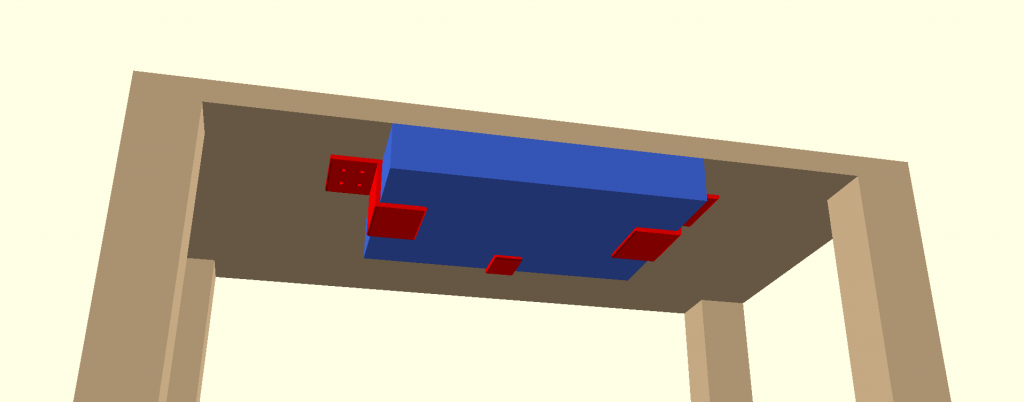In this picture, you can see a device mounted on the underside of a table using red colored brackets. They are exactly what we’re going to create today. Their shape and dimensions will be different for each device. For smaller and lighter devices, we require only a small and light bracket. Bigger things will require a more robust shape and probably also holes for screws, that will hold the brackets in place. We will need all shapes and sizes.

This is a typical task for OpenSCAD’s parametric design. So let’s take a look how to create a single model and modify it by changing a few variables.

Please note that throughout the article, I’m explaining various pieces of code separately to save space. If you want to see the complete code, either scroll to the last two chapters or download the sample code from the last paragraph.

### Round cube

One of the most used constructs when designing models is a cube with rounded corners. Typically, this is generated in OpenSCAD using a ‘hull’ function. This function will create an object that tightly encompasses all of its child objects.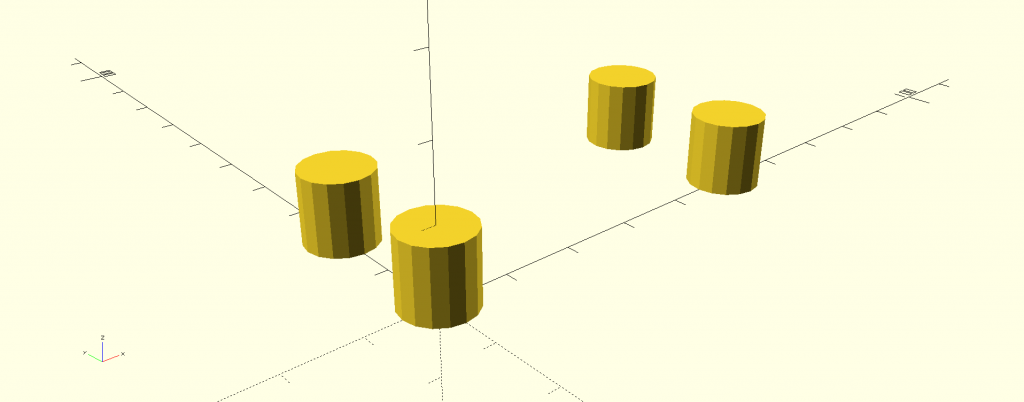These four cylinders will be the base for our cuboid. When we apply the ‘hull’ function, we will get the required shape.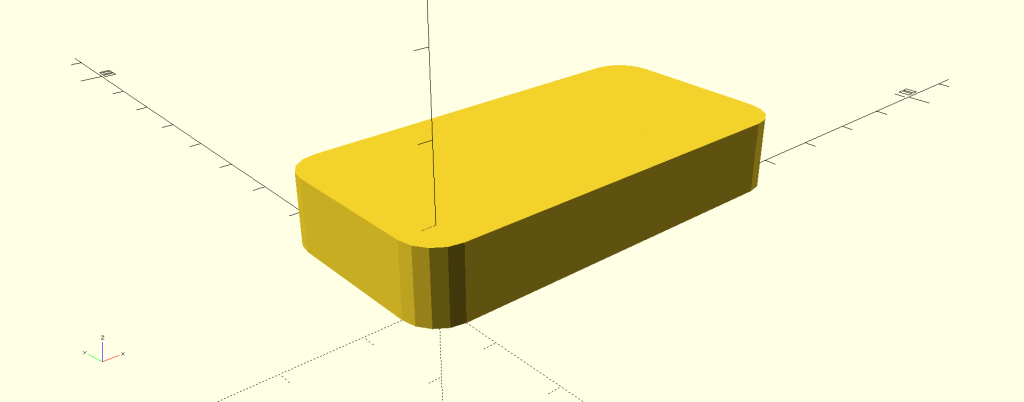However, if we wanted to have rounded corners not only in the projection view but on all edges, then we would have to use eight spheres instead of four cylinders (two spheres in each corner). But in this case, cylinders will be perfectly fine. And since we will be using this shape regularly, we will write a module (this is OpenSCAD’s term for something that’s usually called a method in other programming languages), which will create a cuboid with dimensions and edge curvature based on variables we enter.

The module will be called ‘rcube’ and its code is below. However, at this point, it won’t do anything when you copy-paste it into OpenSCAD, we need to add a bit more.

```module rcube(size, radius) {
hull() {
}
}
```

This module works the same way as the ‘cube’ command, however, it takes into account one more parameter – curvature of rounded edges.

Sometimes, when we create more complex objects, we need to have different curvature of rounded edges in each corner. That’s easy – the ‘radius’ parameter will be a vector containing four different curvature values, as follows:

```module rcube(size, radius) {
hull() {
}
}

```

You can create the following shape by calling ‘rcube([100,50,10], [5,10,20]);’ (without quotes) at the end of the code above: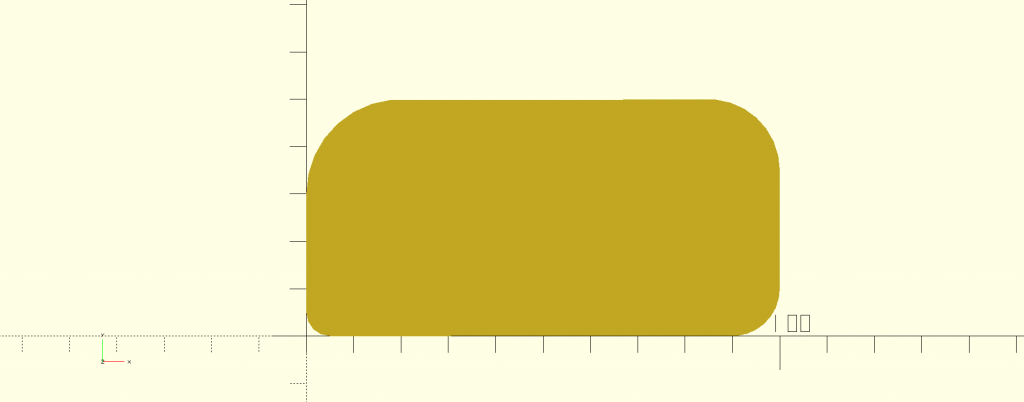Our module is quite good now, but in the current stage, it won’t be able to take into account the fact that some corners have to be sharp. If you enter ‘0’ as the curvature value, the respective corner will be ignored. Let’s modify the code, so when you enter ‘0’, it will create a cube [1,1,n] instead of a cylinder.

```module rcube(size, radius) {
hull() {
// BL
if(radius == 0) cube([1, 1, size]);
// BR
if(radius == 0) translate([size - 1, 0]) cube([1, 1, size]);
// TR
if(radius == 0) translate([size - 1, size - 1])cube([1, 1, size]);
// TL
if(radius == 0) translate([0, size - 1]) cube([1, 1, size]);
}
}

```

The ‘rcube([100, 50, 10], [15, 0, 15, 0]);’ code will now generate a cuboid with two sharp corners and two rounded corners: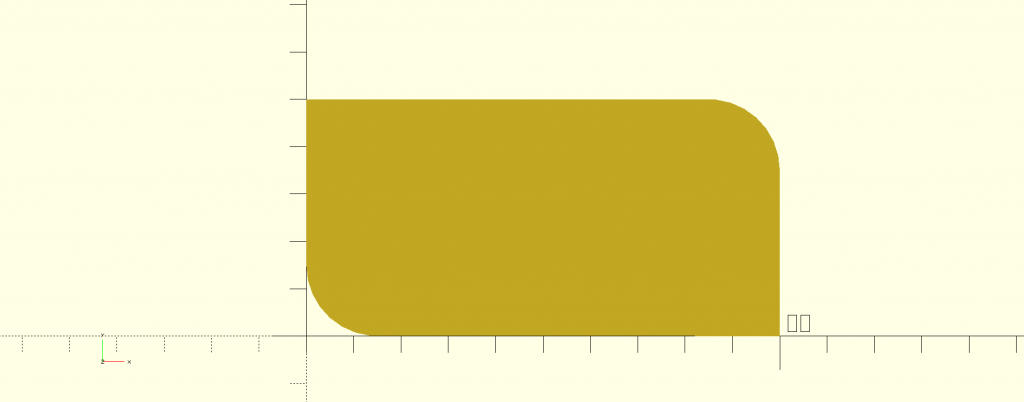Alright, now let’s focus on making the code more user-friendly, mainly because the users will be, in fact, usually us. It would be great if the ‘radius’ parameter was universal.

• If it is a simple variable (a scalar), it determines the radius of a curvature, which is the same for all corners
• If it is a two-component vector, the first number is the curvature of the front corners, the second number is the curvature of the back corners
• If it is a four-component vector, the numbers determine the curvature of all corners, starting with the front left corner and continuing with other corners counter-clockwise

We can find out the length of a vector by using the ‘len()’ function. If a scalar is detected, the returned value will be undef, otherwise, the length of the vector will be returned. We can utilize this, so when the length is not 4, the module will call itself with corresponding parameters.

```module rcube(size, radius) {
// The same radius on all corners
} else if(len(radius) == 2) {
// Different radii on top and bottom
} else if(len(radius) == 4) {
// Different radius on different corners
hull() {
// BL
if(radius == 0) cube([1, 1, size]);
// BR
if(radius == 0) translate([size - 1, 0]) cube([1, 1, size]);
// TR
if(radius == 0) translate([size - 1, size - 1])cube([1, 1, size]);
// TL
if(radius == 0) translate([0, size - 1]) cube([1, 1, size]);
}
} else {
echo("ERROR: Incorrect length of 'radius' parameter. Expecting integer or vector with length 2 or 4.");
}
}
```

### Creating an S-bracket

Why do we care about rounded cubes so much? Well, when you look at the S-bracket closer, you will realize that it actually consists of three cubes (two of them with rounded corners) – it’s nicely visible in the picture below: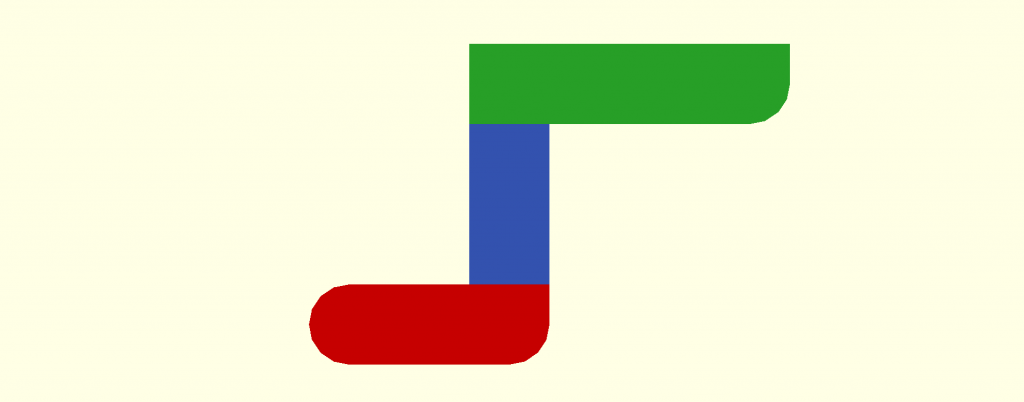So, using the latest version of the ‘rcube’ module from the previous chapter, we can create a parametric design using this code (which needs to be placed at the start of the code from the last chapter):

```width = 30;
height = 30;
top_length = 30;
bottom_length = 20;
number_of_holes = 2;
wall_thickness = 3;
hole_diameter = 4;

\$fn = 16;

rcube([bottom_length + wall_thickness, wall_thickness, width], [wall_thickness / 2, wall_thickness / 2, 0, wall_thickness / 2]);
translate([bottom_length, wall_thickness]) cube([wall_thickness, height, width]);
translate([bottom_length, height]) rcube([top_length + wall_thickness, wall_thickness, width], [0, wall_thickness / 2, 0, 0]);
```

Merging both pieces of code will give you the following result: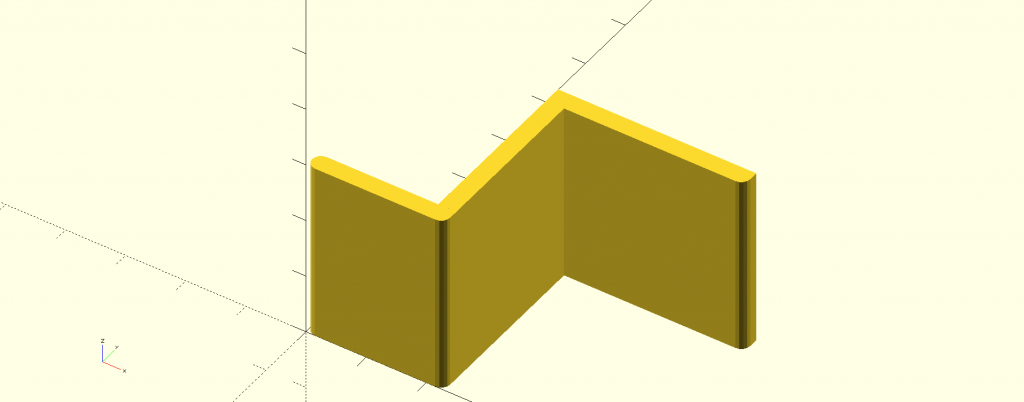The bracket looks good and we could actually leave it in this shape – if we wanted to glue it to the underside of the table – which is often perfectly suitable solution. However, if we want to attach it using screws, we need to make some holes first.

But how many? For smaller brackets, one screw can be enough. Two is better, though, so the bracket doesn’t rotate around the screw. On the other hand, heavier objects will need a sturdier bracket with more holes for at least three to six screws. This means that our bracket needs to have a variable number of holes, let’s say from zero to six, as seen in the picture below.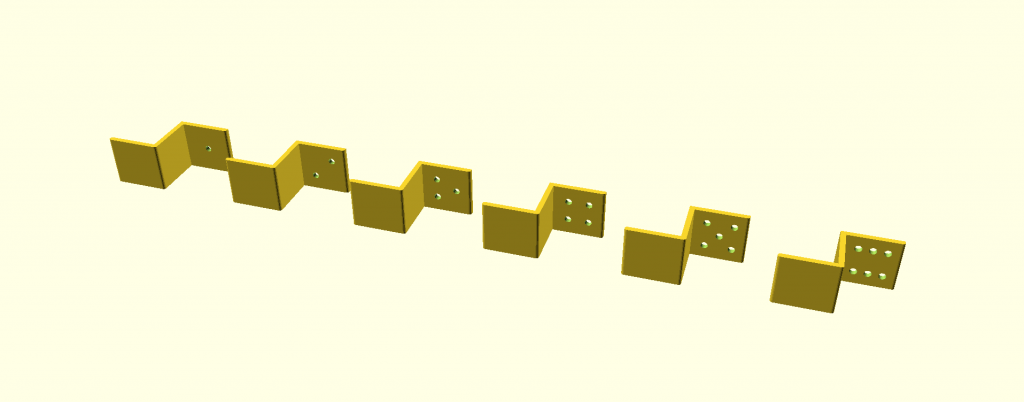Basically, it’s the same problem we solved with the ‘rcube’ module when we had to decide, based on the number of vector components, what will be determined by the values. The code for S-bracket generator (without the ‘rcube’ module code) looks like this:

```width = 30;
height = 30;
top_length = 30;
bottom_length = 20;
number_of_holes = 2;
wall_thickness = 10;
hole_diameter = 4;

fudge = 1;
\$fn = 16;

rcube([bottom_length + wall_thickness, wall_thickness, width], [wall_thickness / 2, wall_thickness / 2, 0, wall_thickness / 2]);
translate([bottom_length, wall_thickness]) cube([wall_thickness, height, width]);
translate([bottom_length, height]) difference() {
rcube([top_length + wall_thickness, wall_thickness, width], [0, wall_thickness / 2, 0, 0]);
if(number_of_holes == 0) {
} else if(number_of_holes == 1) {
// Single centered hole
translate([wall_thickness + top_length * .50, -fudge, width * .50]) rotate([-90, 0, 0]) cylinder(d = hole_diameter, h = wall_thickness + 2 * fudge);
} else if(number_of_holes == 2) {
// Two diagonal holes
translate([wall_thickness + top_length * .30, -fudge, width * .30]) rotate([-90, 0, 0]) cylinder(d = hole_diameter, h = wall_thickness + 2 * fudge);
translate([wall_thickness + top_length * .70, -fudge, width * .70]) rotate([-90, 0, 0]) cylinder(d = hole_diameter, h = wall_thickness + 2 * fudge);
} else if(number_of_holes == 3) {
// Three holes in traingle
translate([wall_thickness + top_length * .30, -fudge, width * .30]) rotate([-90, 0, 0]) cylinder(d = hole_diameter, h = wall_thickness + 2 * fudge);
translate([wall_thickness + top_length * .30, -fudge, width * .70]) rotate([-90, 0, 0]) cylinder(d = hole_diameter, h = wall_thickness + 2 * fudge);
translate([wall_thickness + top_length * .70, -fudge, width * .50]) rotate([-90, 0, 0]) cylinder(d = hole_diameter, h = wall_thickness + 2 * fudge);
} else if(number_of_holes == 4) {
// Four holes in corners
translate([wall_thickness + top_length * .30, -fudge, width * .30]) rotate([-90, 0, 0]) cylinder(d = hole_diameter, h = wall_thickness + 2 * fudge);
translate([wall_thickness + top_length * .70, -fudge, width * .70]) rotate([-90, 0, 0]) cylinder(d = hole_diameter, h = wall_thickness + 2 * fudge);
translate([wall_thickness + top_length * .30, -fudge, width * .70]) rotate([-90, 0, 0]) cylinder(d = hole_diameter, h = wall_thickness + 2 * fudge);
translate([wall_thickness + top_length * .70, -fudge, width * .30]) rotate([-90, 0, 0]) cylinder(d = hole_diameter, h = wall_thickness + 2 * fudge);
} else if(number_of_holes == 5) {
// Four holes in corners and one in cender
translate([wall_thickness + top_length * .25, -fudge, width * .25]) rotate([-90, 0, 0]) cylinder(d = hole_diameter, h = wall_thickness + 2 * fudge);
translate([wall_thickness + top_length * .75, -fudge, width * .75]) rotate([-90, 0, 0]) cylinder(d = hole_diameter, h = wall_thickness + 2 * fudge);
translate([wall_thickness + top_length * .25, -fudge, width * .75]) rotate([-90, 0, 0]) cylinder(d = hole_diameter, h = wall_thickness + 2 * fudge);
translate([wall_thickness + top_length * .75, -fudge, width * .25]) rotate([-90, 0, 0]) cylinder(d = hole_diameter, h = wall_thickness + 2 * fudge);
translate([wall_thickness + top_length * .50, -fudge, width * .50]) rotate([-90, 0, 0]) cylinder(d = hole_diameter, h = wall_thickness + 2 * fudge);
} else if(number_of_holes == 6) {
// Six holes
translate([wall_thickness + top_length * .25, -fudge, width * .25]) rotate([-90, 0, 0]) cylinder(d = hole_diameter, h = wall_thickness + 2 * fudge);
translate([wall_thickness + top_length * .50, -fudge, width * .25]) rotate([-90, 0, 0]) cylinder(d = hole_diameter, h = wall_thickness + 2 * fudge);
translate([wall_thickness + top_length * .75, -fudge, width * .25]) rotate([-90, 0, 0]) cylinder(d = hole_diameter, h = wall_thickness + 2 * fudge);
translate([wall_thickness + top_length * .25, -fudge, width * .75]) rotate([-90, 0, 0]) cylinder(d = hole_diameter, h = wall_thickness + 2 * fudge);
translate([wall_thickness + top_length * .50, -fudge, width * .75]) rotate([-90, 0, 0]) cylinder(d = hole_diameter, h = wall_thickness + 2 * fudge);
translate([wall_thickness + top_length * .75, -fudge, width * .75]) rotate([-90, 0, 0]) cylinder(d = hole_diameter, h = wall_thickness + 2 * fudge);
} else {
echo("ERROR: Unsupported number of holes (supported 0-6");
}
}
```

And that’s pretty much it. Just add the ‘rcube’ module code and try the preview in OpenSCAD. However, I want to show you how to easily modify the code even further.

### Further modifications

In the previous chapter, we have finished our work on the S-bracket generator, which will be good enough for daily use. But we can tweak it a bit more. Personally, I recommend moving the bracket generator into a separate module, so we can call it with additional parameters. Let’s say we want to generate multiple brackets at the same time, however, we want each to have a different number of holes. Once we have the generator in a separate module called ‘bracket’ (see the code below), we can create the bracket at the position we set with the ‘translate’ command and give it a required number of holes. This is just an example of how to modify the code further, you will most likely be fine with the code from the previous chapter.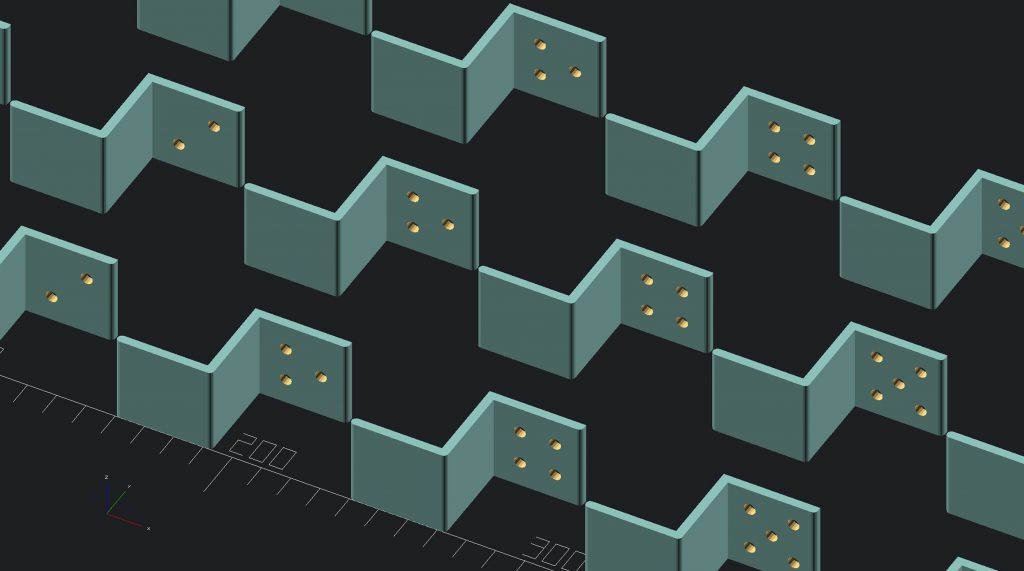```fudge = 1;
\$fn = 16;

translate([000, 0]) bracket(30, 30, 30, 30, number_of_holes = 1);
translate([080, 0]) bracket(30, 30, 30, 30, number_of_holes = 2);
translate([160, 0]) bracket(30, 30, 30, 30, number_of_holes = 3);
translate([240, 0]) bracket(30, 30, 30, 30, number_of_holes = 4);
translate([320, 0]) bracket(30, 30, 30, 30, number_of_holes = 5);
translate([400, 0]) bracket(30, 30, 30, 30, number_of_holes = 6);

module bracket(width, height, top_length, bottom_length, number_of_holes = 2, wall_thickness = 3, hole_diameter = 4) {
rcube([bottom_length + wall_thickness, wall_thickness, width], [wall_thickness / 2, wall_thickness / 2, 0, wall_thickness / 2]);
translate([bottom_length, wall_thickness]) cube([wall_thickness, height, width]);
translate([bottom_length, height]) difference() {
rcube([top_length + wall_thickness, wall_thickness, width], [0, wall_thickness / 2, 0, 0]);
if(number_of_holes == 0) {
} else if(number_of_holes == 1) {
// Single centered hole
translate([wall_thickness + top_length * .50, -fudge, width * .50]) rotate([-90, 0, 0]) cylinder(d = hole_diameter, h = wall_thickness + 2 * fudge);
} else if(number_of_holes == 2) {
// Two diagonal holes
translate([wall_thickness + top_length * .30, -fudge, width * .30]) rotate([-90, 0, 0]) cylinder(d = hole_diameter, h = wall_thickness + 2 * fudge);
translate([wall_thickness + top_length * .70, -fudge, width * .70]) rotate([-90, 0, 0]) cylinder(d = hole_diameter, h = wall_thickness + 2 * fudge);
} else if(number_of_holes == 3) {
// Three holes in traingle
translate([wall_thickness + top_length * .30, -fudge, width * .30]) rotate([-90, 0, 0]) cylinder(d = hole_diameter, h = wall_thickness + 2 * fudge);
translate([wall_thickness + top_length * .30, -fudge, width * .70]) rotate([-90, 0, 0]) cylinder(d = hole_diameter, h = wall_thickness + 2 * fudge);
translate([wall_thickness + top_length * .70, -fudge, width * .50]) rotate([-90, 0, 0]) cylinder(d = hole_diameter, h = wall_thickness + 2 * fudge);
} else if(number_of_holes == 4) {
// Four holes in corners
translate([wall_thickness + top_length * .30, -fudge, width * .30]) rotate([-90, 0, 0]) cylinder(d = hole_diameter, h = wall_thickness + 2 * fudge);
translate([wall_thickness + top_length * .70, -fudge, width * .70]) rotate([-90, 0, 0]) cylinder(d = hole_diameter, h = wall_thickness + 2 * fudge);
translate([wall_thickness + top_length * .30, -fudge, width * .70]) rotate([-90, 0, 0]) cylinder(d = hole_diameter, h = wall_thickness + 2 * fudge);
translate([wall_thickness + top_length * .70, -fudge, width * .30]) rotate([-90, 0, 0]) cylinder(d = hole_diameter, h = wall_thickness + 2 * fudge);
} else if(number_of_holes == 5) {
// Four holes in corners and one in cender
translate([wall_thickness + top_length * .25, -fudge, width * .25]) rotate([-90, 0, 0]) cylinder(d = hole_diameter, h = wall_thickness + 2 * fudge);
translate([wall_thickness + top_length * .75, -fudge, width * .75]) rotate([-90, 0, 0]) cylinder(d = hole_diameter, h = wall_thickness + 2 * fudge);
translate([wall_thickness + top_length * .25, -fudge, width * .75]) rotate([-90, 0, 0]) cylinder(d = hole_diameter, h = wall_thickness + 2 * fudge);
translate([wall_thickness + top_length * .75, -fudge, width * .25]) rotate([-90, 0, 0]) cylinder(d = hole_diameter, h = wall_thickness + 2 * fudge);
translate([wall_thickness + top_length * .50, -fudge, width * .50]) rotate([-90, 0, 0]) cylinder(d = hole_diameter, h = wall_thickness + 2 * fudge);
} else if(number_of_holes == 6) {
// Six holes
translate([wall_thickness + top_length * .25, -fudge, width * .25]) rotate([-90, 0, 0]) cylinder(d = hole_diameter, h = wall_thickness + 2 * fudge);
translate([wall_thickness + top_length * .50, -fudge, width * .25]) rotate([-90, 0, 0]) cylinder(d = hole_diameter, h = wall_thickness + 2 * fudge);
translate([wall_thickness + top_length * .75, -fudge, width * .25]) rotate([-90, 0, 0]) cylinder(d = hole_diameter, h = wall_thickness + 2 * fudge);
translate([wall_thickness + top_length * .25, -fudge, width * .75]) rotate([-90, 0, 0]) cylinder(d = hole_diameter, h = wall_thickness + 2 * fudge);
translate([wall_thickness + top_length * .50, -fudge, width * .75]) rotate([-90, 0, 0]) cylinder(d = hole_diameter, h = wall_thickness + 2 * fudge);
translate([wall_thickness + top_length * .75, -fudge, width * .75]) rotate([-90, 0, 0]) cylinder(d = hole_diameter, h = wall_thickness + 2 * fudge);
} else {
echo("ERROR: Unsupported number of holes (supported 0-6");
}
}
}

// The same radius on all corners
} else if(len(radius) == 2) {
// Different radii on top and bottom
} else if(len(radius) == 4) {
// Different radius on different corners
hull() {
// BL
if(radius == 0) cube([1, 1, size]);
// BR
if(radius == 0) translate([size - 1, 0]) cube([1, 1, size]);
// TR
if(radius == 0) translate([size - 1, size - 1])cube([1, 1, size]);
// TL
if(radius == 0) translate([0, size - 1]) cube([1, 1, size]);